# Monte Carlo methods for risk analysis Stochastic simulation and numerical experiments

## OverviewSome problems in risk analysis cannot be expressed in an analytical form. Others are difficult to define in a deterministic manner. Monte Carlo methods (also known as stochastic simulation techniques) consist of running “numerical experiments” to observe what happens “on average” over a large number of runs of a stochastic model. They involve repeated random sampling from input probability distributions, execution of the model with these stochastic inputs, then aggregation of the large number of executions to obtain an estimate of the quantity of interest. In some cases Monte Carlo methods are a simple and convenient alternative to analytical methods, allowing you to express your problem in a direct way and let the computer do the hard work. For complex problems which cannot be resolved analytically, they may be the only way of estimating the output of interest. These methods rely on the speed of modern computers and their ability to generate pseudo-random numbers from various relevant probability distributions.

Monte Carlo methods are widely used in risk analysis, for instance for:

• propagating uncertainty through a numerical model to obtain confidence intervals on your model outputs

• estimating quantile measures for performance measures

• simulating evacuation from a building during the design phase

• predicting failure, cost overruns and schedule overruns in project management

This submodule is a part of the risk analysis module.

## Course material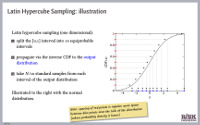Monte Carlo methods in risk analysisLecture slides (PDF)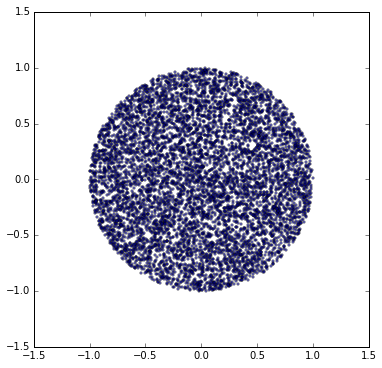Estimating pi using a Monte Carlo simulationDownload Python notebookRun notebook in MyBinderRun notebook in Google Colab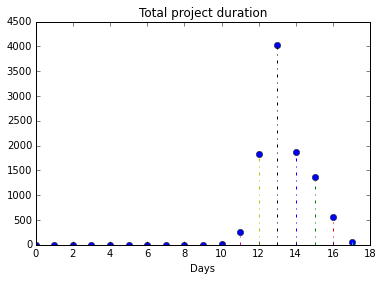Project risk analysis with Monte Carlo simulationDownload Python notebookRun notebook in MyBinderRun notebook in Google Colab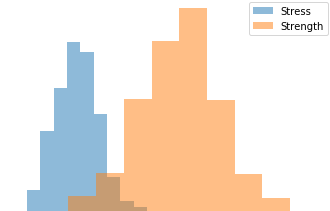Monte Carlo simulation of failure probability in mechanical designDownload Python notebookRun notebook in MyBinderRun notebook in Google ColabEstimating failure probability of a space vessel fuel tankDownload Python notebookRun notebook in MyBinderRun notebook in Google ColabEstimating failure probability of a space vessel fuel tank (Rust kernel)View Rust notebook onlineDownload Rust notebook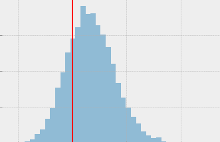Monte Carlo simulation of landslide riskDownload Python notebookRun notebook in MyBinderRun notebook in Google Colab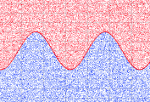Numerical integration using Monte Carlo methodsDownload Python notebookRun notebook in MyBinderRun notebook in Google Colab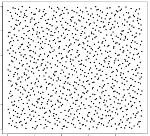Monte Carlo sampling methodsDownload Python notebookRun notebook in MyBinderRun notebook in Google Colab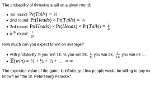Limits of stochastic simulation methods: the Saint Petersburg problemDownload Python notebookRun notebook in MyBinderRun notebook in Google ColabVarious poker odds: working with discrete probability distributionsDownload Python notebookRun notebook in MyBinderRun notebook in Google ColabIn these course materials, applications are presented using the NumPy, SciPy and statsmodels libraries for the Python programming language. We have some material on getting started with Python that explains how to install Python on your computer or try out our computational notebooks using free online services.

One of the notebooks above shows how to implement Monte Carlo sampling in a notebook-style interactive environment with the high-performance Rust programming language, which will typically be many thousands of times faster than implementations in Python. We also include links to relevant plugins for Microsoft Excel, if you’re into that kind of thing.

One of the Python notebooks above illustrates alternative methods to standard (naïve) random sampling:

• Latin Hypercube Sampling (LHS) or stratified sampling without replacement involves splitting up each input variable into a number of equiprobable intervals and sampling separately from each interval, using the inverse cumulative distribution function for that variable. In typically leads to faster convergence than Monte Carlo procedures that implement standard random sampling.

• Low-discrepancy sequences or quasi-random sequences such as the Halton and Sobol’ sequences, which fill the input space more quickly than pseudorandom sequences and typically lead to faster convergence than standard random sampling. These are based on deterministic mathematical sequences of points that don’t “cluster” together.

The notebook on stochastic simulation of the Saint Petersburg problem linked to above illustrates a situation where very unlikely events have an extremely high impact on the mean outcome. In such situations, Monte Carlo simulation is not a good approach to estimate the output of interest (expected value of playing the game, in this case).

## Other resources

We recommend the following sources of further information on this topic:

Published:
Last updated: